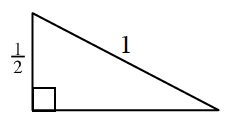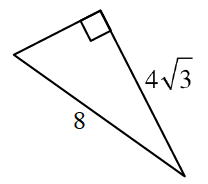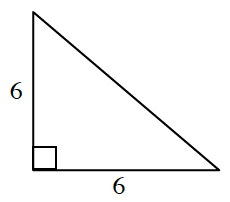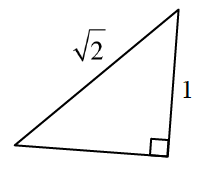### Home > INT3 > Chapter 8 > Lesson 8.1.3 > Problem8-53

8-53.

Calculate the length of the missing side in each triangle below. Write your answers in exact form.

1.1.1.1.Recall the side length ratios of the Special Right Triangles (from a previous course) or use the Pythagorean Theorem.

$4$

$1$